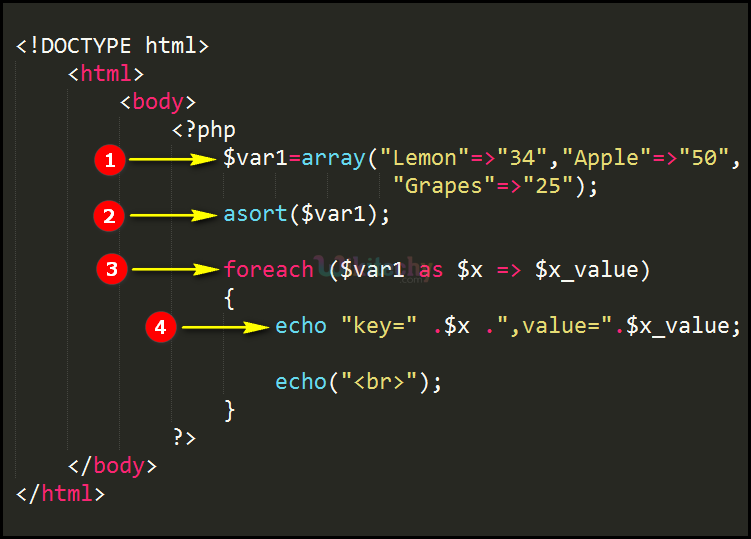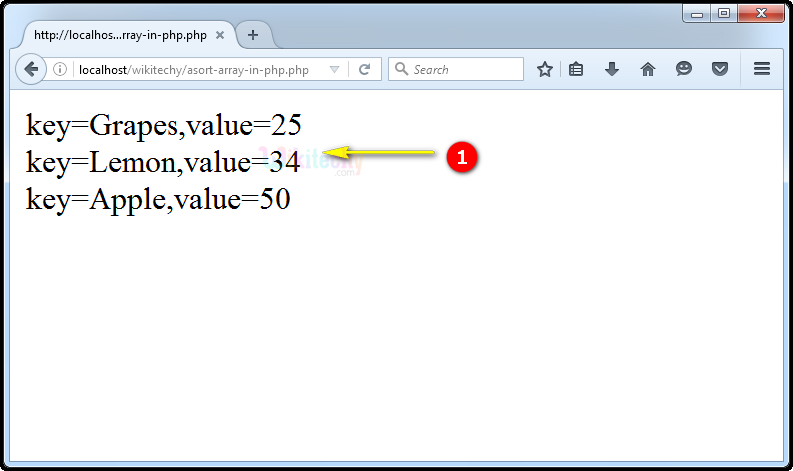# php tutorial - PHP asort Function - php programming - learn php - php code - php script

• In PHP, asort function is mainly used in sorting associative arrays.
• The "asort()" function is used to sort an array elements by its array values by keeping its keys as it is.

## php examples Code Explanation :1. In this statement “\$var1” variable is defining an associative array using array function with its keys “Lemon, Apple and Grape” and the values are “34,50, and 25”.
2. The var1 value will be sorted using the asort function where the array values are sorted in ascending order.
3. In this statement we use the for each loop that will be used for looping process in an associative array. The first variable of the for loop defines the array name “\$var1” then “\$x” defines the key, and “\$x_value” defines the value.
4. Echo statement is used for printing the value sorted key and their values. Whereas the “.” Dot symbol is used for concatenation of the string in php.

## php tutorials Sample Output :1. Output -> the key values (34, 50 and 25) are sorted in ascending order (25 ,34 and 50) by keeping its keys (Grapes, Lemon & Apple) as it is.